’╗┐ Varipend┬«contents:

Contacts

Engl

Find: in
About an opportunity of "reactionless" moving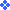2. Article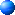2.4. Conclusions

Calculation of moving of the center of mass of the closed mechanical system is executed on the basis of a principle of conservation of momentum and by means of Lagrange's equations.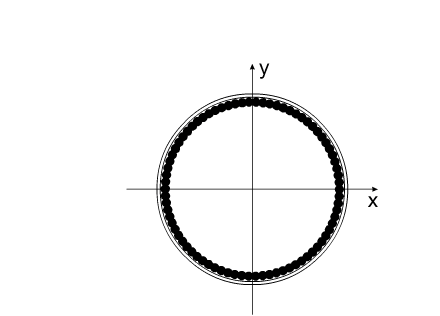In time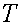the total momentum of system is equal to zero (Fig. 8).  Moving all closed system arises only during the working period.

In a Fig. 8 plots of change of momentums for the working period are presented:

systems of mobile elements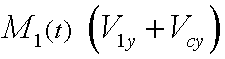and "motionless", cases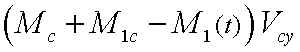The plot of a total momentum, i.e. linear momentum of all system, coincides with an axis of abscissas the plot.

If at any moment to stop relative moving system of mobile elements, all mechanical system will have initial speed.

If during the initial moment of time all mechanical system had zero speed in system XOY, that after a stop of system of mobile elements speed of all mechanical system will be equaled also to zero!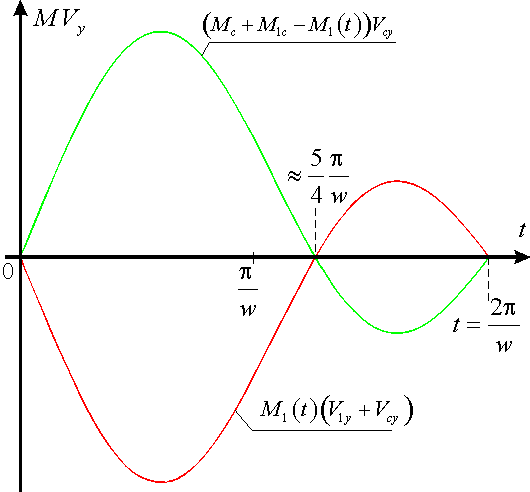Fig. 8

It is possible to speak only about conditional speed and conditional acceleration of the center of mass of all mechanical system for the working period.

Speed of system is a derivative on time from coordinate.

Let's leave while in the party calculation on one of coordinates. Below it will be explained, in this connection it is connected.

The greatest interest represents change of coordinate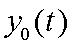(25) or (31).(34)(35)

Corresponding plots of change of speed and acceleration of all closed mechanical system are presented in Fig. 9 and Fig. 10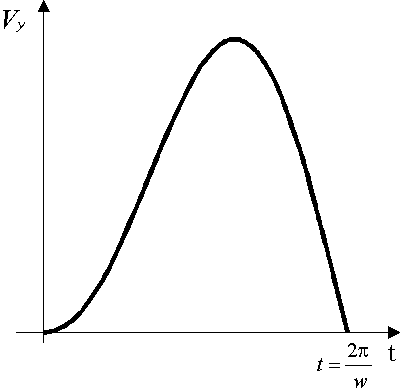Fig.9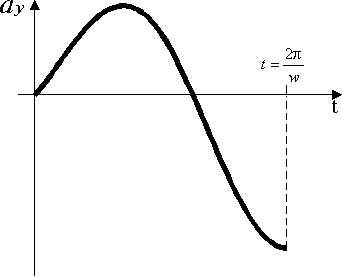Fig. 10

// //

ąĪą░ą╣čé čüąŠąĘą┤ą░ąĮ ą▓ čüąĖčüčéąĄą╝ąĄ uCoz